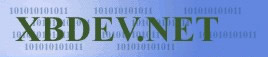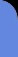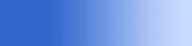Tuesday July 16, 2019
 home | about | contact | DonationsXNA & 360 The bits and bytes of XNA and C# for your 360...

Object Picking

This is when we want to take a 2D point on screen and create a ray in your 3D world, one from your near plane to your far plane.  This ray/line segment is used to pick various objects in our world.  Either using the mouse or some other 2d cursor move around the screen using the controller.

There are various ways of doing this, below I've shown 3 different way of doing it, just so you can see that there's more than one way to cook an egg ;)

• Invert View/Projection Matrix and multiply this by our 2D point

• Use the built in Unproject API (which basically does the inverse and multiplication for us)

• Extract the data from the View matrix and project a ray into the screen.

First I'll give a snippet of the camera where setting up - just so you can see where using a simple projection and lookat camera - of course the principles should work with different projection/orthogonal setups.

 Snippet - Setting up camera // Setup a basic camera view and projection for testing float aspectRatio = (float)m_device.Viewport.Width / m_device.Viewport.Height;   Matrix camView = Matrix.CreateLookAt(new Vector3(0,5,5),                     Vector3.Zero,                     Vector3.Up);        float nearPlaneDist = 1.0f; float farPlaneDist  = 1000.0f;   Matrix camProj = Matrix.CreatePerspectiveFieldOfView(MathHelper.ToRadians(45.0f),                                     aspectRatio,                                     nearPlaneDist,                                     farPlaneDist);

First we just use the inverse of our projection matrix - probably not the best way as where going to a lot of work by finding the inverse of our projection and view - but it goes to show that the opposite of what our matrix does can give us what we started with.

 Snippet // Pixel Space - ScreenSpace -1 to 1 Vector2 screenSpace; screenSpace.X =  ( ( ( 2.0f * mousePos.X ) / screenSize.X  ) - 1 ); screenSpace.Y = -( ( ( 2.0f * mousePos.Y ) / screenSize.Y  ) - 1 );   // Inverse View/Proj Matrix Matrix invMProj = Matrix.Invert(camProj); Matrix invMView = Matrix.Invert(camView);   // Near/Far Point Vector3 nearPos = new Vector3(screenSpace.X, screenSpace.Y, 0); Vector3 farPos  = new Vector3(screenSpace.X, screenSpace.Y, 1);   // Final near and far ray positions nearPos = Vector3.Transform(nearPos, invMProj * invMView)   * nearPlaneDist; farPos  = Vector3.Transform(farPos,  invMProj * invMView)   * farPlaneDist;

A more optimised way is using the build in API one - just to note, in C++ and DirectX theres a D3DXVec3Unproject(..) as well.  MS is always trying to help us out.  The near and far world points should be the same as what we got above using our own matrix.

 Snippet // Alternative Way! Vector3 nearScreenPoint = new Vector3(mousePos.X, mousePos.Y, 0); Vector3 farScreenPoint  = new Vector3(mousePos.X, mousePos.Y, 1); Vector3 nearWorldPoint  = m_device.Viewport.Unproject(nearScreenPoint, projection, view, Matrix.Identity); Vector3 farWorldPoint   = m_device.Viewport.Unproject(farScreenPoint,  projection, view, Matrix.Identity);

Finally - using what our camera actually holds, we can extract a starting point and a ray direction.

 Snippet // Another way again Vector3 v; v.X = -( ( ( 2.0f * mousePos.X ) / screenSize.X  ) - 1 ) / camProj.M11; v.Y =  ( ( ( 2.0f * mousePos.Y ) / screenSize.Y  ) - 1 ) / camProj.M22; v.Z =  1.0f;   Matrix m = Matrix.Invert(camView); Vector3 rayOrigin = new Vector3(m.M41, m.M42, m.M43); m.M41 = m.M42 = m.M43 = 0.0f; Vector3 rayDir    = Vector3.Transform(v, m);   // We have a ray origin and ray dir - but we can create a near and far // as we have with the others as: Vector3 nearPos = rayOrigin; Vector3 farPos  = rayOrigin - Vector3.Normalize(rayDir)*1000.0f;

 Visitor: 9534626  { 209.237.238.175 } Copyright (c) 2002-2017 xbdev.net - All rights reserved. Designated tutorial and software are the property of their respective owners.